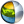Cg 3.1 Toolkit Documentation Cg / Standard Library / frexp

## Name

frexp - splits scalars and vectors into normalized fraction and a power of 2

## Synopsis

```float  frexp(float x, out float e);
float1 frexp(float1 x, out float1 e);
float2 frexp(float2 x, out float2 e);
float3 frexp(float3 x, out float3 e);
float4 frexp(float4 x, out float4 e);

half   frexp(half x, out half e);
half1  frexp(half1 x, out half1 e);
half2  frexp(half2 x, out half2 e);
half3  frexp(half3 x, out half3 e);
half4  frexp(half4 x, out half4 e);

fixed  frexp(fixed x, out fixed e);
fixed1 frexp(fixed1 x, out fixed1 e);
fixed2 frexp(fixed2 x, out fixed2 e);
fixed3 frexp(fixed3 x, out fixed3 e);
fixed4 frexp(fixed4 x, out fixed4 e);
```

## Parameters

x
Vector or scalar of which to split.
e
Vector or scalar where the exponent of x is output.

## Description

This function decomposes x into two parts: a mantissa between 0.5 and 1 (returned by the function) and an exponent output as e.

If the value x is zero, both parts of the result are zero.

For vectors, the returned vector contains the mantissa of each element of the input vector and the output vector contains the exponent of each element of the input vector.

## Reference Implementation

The example below is not legal Cg because it uses the & address-of operator not supported by Cg in order to call the ANSI C frexp routine.

```float3 frexp(float3 x, out float3 e)
{
float3 rv;
int i;

for (i=0; i<3; i++) {
float eout;

rv[i] = frexp(a[i], &eout);  // this is the ANSI C standard library frexp()
e[i] = eout;
}
return rv;
}
```

## Profile Support

frexp is fully supported in all profiles unless otherwise specified.

Support in the fp20 is limited to constant compile-time evaluation.| Cg Toolkit | Download | Release Archive | Profiles | Reference | Books | Discussions |